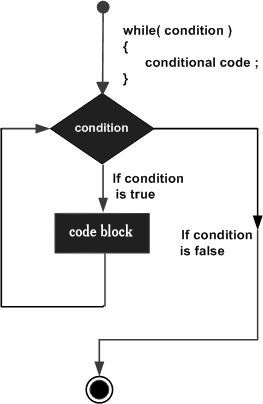# D Programming - While Loop

A while loop statement in D programming language repeatedly executes a target statement as long as a given condition is true.

## Syntax

The syntax of a while loop in D programming language is −

```while(condition) {
statement(s);
}
```

Here, statement(s) may be a single statement or a block of statements. The condition may be any expression, and true is any nonzero value. The loop iterates while the condition is true.

When the condition becomes false, program control passes to the line immediately following the loop.

## Flow DiagramHere, key point of the while loop is that the loop might not ever run. When the condition is tested and the result is false, the loop body is skipped and the first statement after the while loop is executed.

## Example

```import std.stdio;

int main () {
/* local variable definition */
int a = 10;

/* while loop execution */
while( a < 20 ) {
writefln("value of a: %d", a);
a++;
}

return 0;
}
```

When the above code is compiled and executed, it produces the following result −

```value of a: 10
value of a: 11
value of a: 12
value of a: 13
value of a: 14
value of a: 15
value of a: 16
value of a: 17
value of a: 18
value of a: 19
```
d_programming_loops.htm